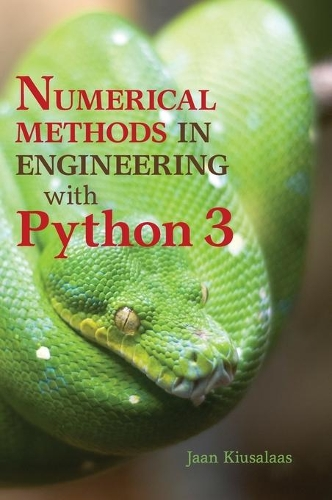•# Numerical Methods in Engineering with Python 3 (Hardback)

(author)
£80.99
Hardback 432 Pages / Published: 21/01/2013
• We can order this from the publisher

Usually dispatched within 15 working days

This book is an introduction to numerical methods for students in engineering. It covers solution of equations, interpolation and data fitting, solution of differential equations, eigenvalue problems and optimisation. The algorithms are implemented in Python 3, a high-level programming language that rivals MATLAB ® in readability and ease of use. All methods include programs showing how the computer code is utilised in the solution of problems. The book is based on Numerical Methods in Engineering with Python, which used Python 2. This new edition demonstrates the use of Python 3 and includes an introduction to the Python plotting package Matplotlib. This comprehensive book is enhanced by the addition of numerous examples and problems throughout.

Publisher: Cambridge University Press
ISBN: 9781107033856
Number of pages: 432
Weight: 930 g
Dimensions: 257 x 183 x 25 mm
Edition: 3rd Revised edition

MEDIA REVIEWS
'... a practical introduction, pushing the theory as far in the background as possible.' The European Mathematical Society (euro-math-soc.eu)
'This book is nicely focused on the most frequently encountered types of numerical problems that scientists and engineers usually face and the most common and robust algorithms for solving them. The text is just the right size for a semester-long course for upper-division undergraduates or first-year graduate students ... this is a well-written text that is logically organized, attractively presented, and supported with challenging problems.' Anthony J. Duben, Computing Reviews